This tutorial illustrates the application of the TropFishR package to perform a single-species fish stock assessment with length frequency (LFQ) data. According to @Sparre1998b, this includes following steps: (1) estimation of biological stock characteristics (growth and natural mortality), (2) exploration of fisheries aspects (fishing mortality rate and selectivity), (3) assessment of stock status. The order of the methods is important as they build upon each other in a sequential way. Data from literature might be used to skip a step in this workflow or to compare them to the outcomes of this routine.

### Installing TropFishR

The current version of TropFishR (v1.6.3) requires R $$>= 3.0.0$$ and can be downloaded from CRAN as follows.

install.packages("TropFishR", repos = "https://cran.rstudio.com/")


Alternatively, the package can be installed from GitHub:

install.packages("remotes")
remotes::install_github("tokami/TropFishR")


The package is loaded into the R environment with:

library(TropFishR)


The tutorial will make use of a synthetic LFQ data set included in the package (“synLFQ7”). We load the data set into the R environment with data(synLFQ7) and rename it with: lfq <- synLFQ7.

### Biological stock characteristics

Growth, natural mortality, recruitment patterns and the stock-recruitment relationship are important biological stock characteristics and input parameters for population dynamics and yield per recruit models.

#### Growth parameters

Commonly used growth parameters are the asymptotic length ($$L_{\infty}$$), the growth coefficient ($$K$$) and the theoretical length at age zero ($$t_{0}$$) of the von Bertalanffy growth function (VBGF). The ELEFAN (ELectronic LEngth Frequency ANalysis) methods allow to estimate $$L_{\infty}$$ and $$K$$ from LFQ data by restructuring the data and fitting growth curves through the restructured LFQ data [@Pauly1980]. I recommend to start by visualising the raw and restructured LFQ data, which aids in determining an appropriate bin size and the moving average of the restructuring procedure. The function lfqModify allows to change the bin size by setting the argument bin_size to a numeric. The function lfqRestructure is used for the restructuring process, where the argument MA allows to control the number of bins used for the moving average and the argument addl.sqrt allows to apply an additional squareroot transformation in the restructuring process, which reduces the weighting of large individuals.

## set seed value for reproducible results
set.seed(1)

## adjust bin size
lfq_bin2 <- lfqModify(lfq, bin_size = 2)

## plot raw and restructured LFQ data
ma <- 7
lfq_bin2_res <- lfqRestructure(lfq_bin2, MA = 7, addl.sqrt = FALSE)

opar <- par(mfrow = c(2,1), mar = c(2,5,2,3), oma = c(2,0,0,0))
plot(lfq_bin2_res, Fname = "catch", date.axis = "modern")
plot(lfq_bin2_res, Fname = "rcounts", date.axis = "modern")
par(opar)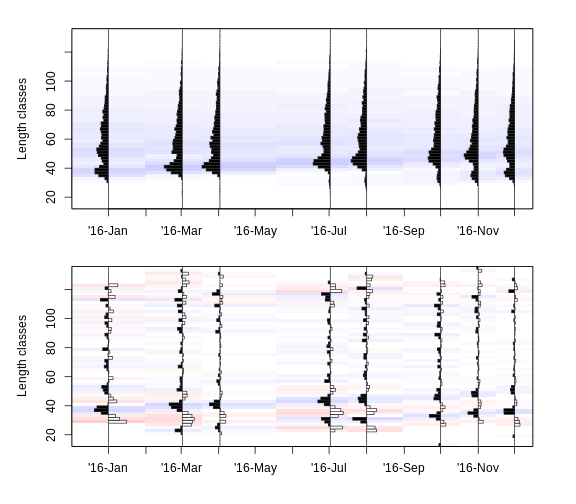Based on visual inspection of the length-frequency distributions, a bin size of 2 cm and a moving average of 7 seems appropriate for this data set and will be used in the following.

In TropFishR, there are 4 different methods based on the ELEFAN functionality: (i) K-Scan for the estimation of K for a fixed value of $$L_{inf}$$, (ii) Response Surface Analysis (RSA), (iii) ELEFAN with simulated annealing (ELEFAN_SA), and (iv) ELEFAN with a genetic algorithm (ELEFAN_GA), where the last three methods all allow to estimate K and $$L_{\infty}$$ simultaneously. The K-Scan method does not allow to test if different combinations of $$L_{\infty}$$ and K might result in a better fit. RSA with a range around $$L_{\infty}$$ can be used to check different combinations. However, RSA can be very slow and does not allow to optimise over the parameters C and $$t_s$$ of the seasonalised VBGF (soVBGF). It only allows to compare the score of ELEFAN runs with manually fixed C and $$t_s$$ values. In contrast, the newly implemented ELEFAN method ELEFAN_SA using a simulated annealing algorithm [@Xiang2013] and ELEFAN_GA using genetic algorithms allow for the optimisation of the soVBGF [@Taylor2016]. The optimisation procedure in the simulated annealing algorithm gradually reduces the stochasticity of the search process as a function of the decreasing “temperature” value, which describes the probability of accepting worse conditions.

Here, we will apply the two new ELEFAN functions ELEFAN_SA and ELEFAN_GA. Both functions require to define the lower (low_par) and upper (up_par) bounds of the search space for all growth parameters. For this data set, we assume a wide search space for $$K$$ and use the maximum length in the data to define the search space for $$L_{\infty}$$ [@Taylor1958; @Beverton1963]. We assume the full parameter space for the other parameters ($$t_{anchor}$$, $$C$$, $$t_s$$).

## coarse estimate of Linf
linf_guess <- max(lfq_bin2$midLengths) / 0.95 ## lower search space bounds low_par <- list(Linf = 0.8 * linf_guess, K = 0.01, t_anchor = 0, C = 0, ts = 0) ## upper search space bounds up_par <- list(Linf = 1.2 * linf_guess, K = 1, t_anchor = 1, C = 1, ts = 1)  Then ELEFAN_SA can be applied as follows. ## run ELEFAN with simulated annealing res_SA <- ELEFAN_SA(lfq_bin2, SA_time = 60*0.5, SA_temp = 6e5, MA = ma, seasonalised = TRUE, addl.sqrt = FALSE, init_par = list(Linf = linf_guess, K = 0.5, t_anchor = 0.5, C=0.5, ts = 0.5), low_par = low_par, up_par = up_par) ## show results res_SA$par
res_SA$Rn_max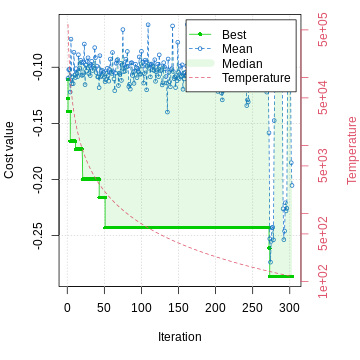Note that the computing time can be controlled with the argument SA_time and the results might change when increasing the time, in case the stable optimum of the objective function was not yet reached (stable optimum is indicated by overlapping blue and green dots in the score graph). Due to the limitations of the vignette format the computation time was set to 0.5 minutes, which results already in acceptable results of $$L_{\infty}$$ = 123.05, K = 0.2, $$t_{anchor}$$ = 0.27, C = 0.38, and $$t_s$$ = 0.42 with a score value ($$Rn_{max}$$) of 0.29. I recommend to increase SA_time to 3 - 5 minutes to increase chances of finding the stable optimum. Another new optimisation routine is based on generic algorithms and is applied by the function ELEFAN_GA. ## run ELEFAN with genetic algorithm res_GA <- ELEFAN_GA(lfq_bin2, MA = ma, seasonalised = TRUE, maxiter = 50, addl.sqrt = FALSE, low_par = low_par, up_par = up_par, monitor = FALSE) ## show results res_GA$par
res_GA$Rn_max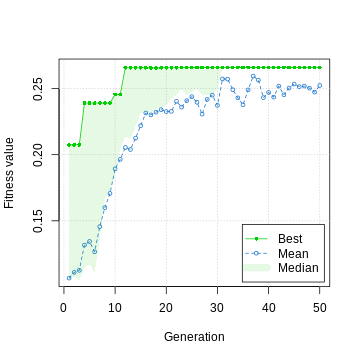The generation number of the ELEFAN_GA was set to only 50 generations (argument maxiter), which returns following results: $$L_{\infty}$$ = 123.79, K = 0.22, $$t_{anchor}$$ = 0.33, C = 0.49, and $$t_s$$ = 0.31 with a score value ($$Rn_{max}$$) of 0.27. As with ELEFAN_SA the generation number was hold down due to the vignette format and should be increased in order to find more stable results. According to [@Pauly1980] it is not possible to estimate $$t_{0}$$ (theoretical age at length zero) from LFQ data alone. However, this parameter does not influence results of the methods of the traditional stock assessment workflow (catch curve and yield per recruit model) and can be set to zero. The ELEFAN methods in this package do not return starting points as FiSAT II users might be used to. Instead, they return the parameter t_anchor, which describes the fraction of the year where yearly repeating growth curves cross length equal to zero; for example a value of 0.25 refers to April 1st of any year. The maximum age is estimated within the ELEFAN function: it is the age when length is 0.95 $$L_{\infty}$$. However, this value can also be fixed with the argument agemax, when alternative information about the maximum age of the fish species is available. The jack knife technique allows to estimate a confidence interval around the parameters of the soVBGF [@Quenouille1956; @Tukey1958; @Tukey1986]. This can be automated in R with following code: ## list for results JK <- vector("list", length(lfq_bin2$dates))

## loop
for(i in 1:length(lfq_bin2$dates)){ loop_data <- list(dates = lfq_bin2$dates[-i],
midLengths = lfq_bin2$midLengths, catch = lfq_bin2$catch[,-i])
tmp <- ELEFAN_GA(loop_data, MA = ma, seasonalised = TRUE,
maxiter = 50, addl.sqrt = FALSE,
low_par = low_par,
up_par = up_par,
monitor = FALSE, plot = FALSE)
JK[[i]] <- unlist(c(tmp$par, list(Rn_max=tmp$Rn_max)))
}

## bind list into dataframe
JKres <- do.call(cbind, JK)

## mean
JKmeans <- apply(as.matrix(JKres), MARGIN = 1, FUN = mean)

## confidence intervals
JKconf <- apply(as.matrix(JKres), MARGIN = 1, FUN = function(x) quantile(x, probs=c(0.025,0.975)))
JKconf <- t(JKconf)
colnames(JKconf) <- c("lower","upper")

## show results
JKconf


Depending on the number of sampling times (columns in the catch matrix) and the maxiter, this loop can take some time as ELEFAN runs several times, each time removing the catch vector of one of the sampling times.

The fit of estimated growth parameters can also be explored visually and indicates high similarity with true growth curves and a good fit through the peaks of this data set.

## plot LFQ and growth curves
plot(lfq_bin2_res, Fname = "rcounts",date.axis = "modern", ylim=c(0,130))
lt <- lfqFitCurves(lfq_bin2, par = list(Linf=123, K=0.2, t_anchor=0.25, C=0.3, ts=0),
draw = TRUE, col = "grey", lty = 1, lwd=1.5)
lt <- lfqFitCurves(lfq_bin2, par = res_SA$par, draw = TRUE, col = "darkblue", lty = 1, lwd=1.5) lt <- lfqFitCurves(lfq_bin2, par = res_GA$par,
draw = TRUE, col = "darkgreen", lty = 1, lwd=1.5)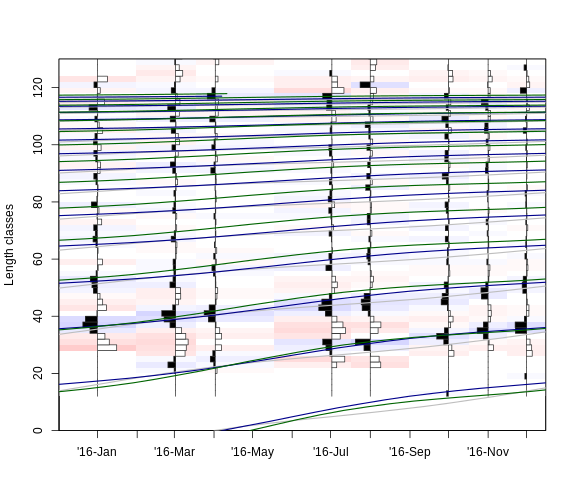For further analysis, we use the outcomes of ELFAN with genetic algorithm by adding them to the Thumbprint Emperor data list.

## assign estimates to the data list
lfq_bin2 <- lfqModify(lfq_bin2, par = res_GA$par)  #### Natural mortality rate The instantaneous natural mortality rate (M) is an influential parameter of stock assessment models and its estimation is challenging [@Kenchington2014; @Powers2014]. When no controlled experiments or tagging data is available the main approach for its estimation is to use empirical formulas. Overall, there are at least 30 different empirical formulas for the estimation of this parameter [@Kenchington2014] relying on correlations with life history parameters and/or environmental information. We apply the most recent formula, which is based upon a meta-analysis of 201 fish species [@Then2015] and assign the results to our data set. This method requires estimates of the VBGF growth parameters [$$L_{\infty}$$ and K; @Then2015]. ## estimation of M Ms <- M_empirical(Linf = lfq_bin2$par$Linf, K_l = lfq_bin2$par$K, method = "Then_growth") ## assign M to data set lfq_bin2$par$M <- as.numeric(Ms)  The result is a natural mortality of 0.28 year$$^{-1}$$. ### Fishing mortality rates and gear selectivity Another prerequisite for the stock status estimation is knowledge on fishing mortality rate (F) (usually derived by subtracting natural mortality from total mortality) and gear selectivity is necessary. The length-converted catch curve allows the estimation of the instantaneous total mortality rate (Z) of LFQ data and the derivation of a selection ogive. Here we skip an in-depth selectivity exploration, because more data would be required for this assessment. For a comprehensive description of selectivity estimation refer to @Millar1997b. The following approach assumes a logistic selection ogive, typical for trawl-net selectivity, which may provide an appropriate first estimate in the case of LFQ data derived from a mixture of gears. The total mortality rate is estimated with a sample of the catch representative for the whole year. Besides, changing the bin size, the function lfqModify allows rearranging the catch matrix in the required format (catch vector per year) and optinal pooling of the largest length classes with only a few individuals into a plus group. ## define plus group as largest length class smaller than Linf plus_group <- lfq_bin2$midLengths[max(which(lfq_bin2$midLengths < lfq_bin2$par$Linf))] ## summarise catch matrix into vector and add plus group lfq_catch_vec <- lfqModify(lfq_bin2, vectorise_catch = TRUE, plus_group = plus_group)  The catch curve can then be applied to the vectorised data set with catchCurve(lfq_catch_vec). This triggers an interactive plotting function where the user selects points to be used in the regression analysis by clickling on the first and last point to be included. Please find for more information with help(catchCurve). Alternatively, the argument reg_int defines the first and last point to be used in the regression analysis and, thus, allows to avoid the interactive plotting function. For this data set, the 18th and 55th point seem to be reasonable first and last points of the regression analysis. The argument calc_ogive allows the estimation of the selection ogive. Note, that you might require the argument catch_columns if your data set spans multiple years as it allows to choose the columns (years after vectorisation) of the catch matrix which will be summarised for the analysis. Here, we do not need this argument as the catch matrix only includes catches from 2016. If data of several years are available, the catch curve can either be applied to the years separately (e.g. catch_columns=1 for the first year), or to the data of several years combined (e.g. catch_columns=c(1,2)). ## run catch curve res_cc <- catchCurve(lfq_catch_vec, reg_int = c(18,55), calc_ogive = TRUE) ## assign estimates to the data list lfq_catch_vec$par$Z <- res_cc$Z
lfq_catch_vec$par$FM <- as.numeric(lfq_catch_vec$par$Z - lfq_catch_vec$par$M)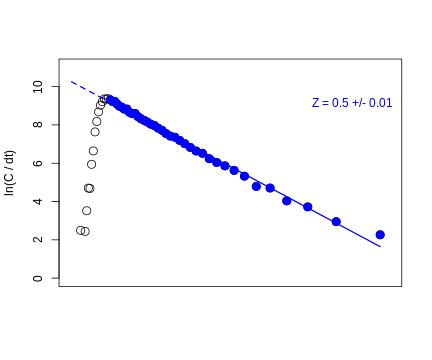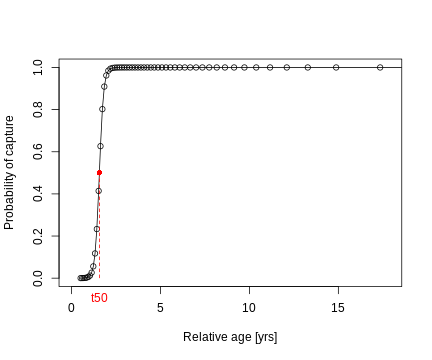The catch curve analysis returns a Z value of 0.5 $$year^{-1}$$. By subtracting M from Z, the fishing mortality rate is derived: 0.22 $$year^{-1}$$. The selectivity function of the catch curve estimated a length at 50% probability of capture ($$L_{50}$$) of 35.81 cm.

### Stock status

#### Exploitation rate

The exploitation rate is defined as $$E = F/Z$$ and relative to the reference level of 0.5, provides a simple indication of the stock status @Gulland1983.

lfq_catch_vec$par$E <- lfq_catch_vec$par$FM / lfq_catch_vec$par$Z


For this data set, the exploitation rate is equal to 0.44 and, thus, does not indicate overfishing.

#### Yield per recruit modelling

Prediction models (or yield per recruit models, e.g. Thompson and Bell model) allow to evaluate the status of a fish stock in relation to reference levels and to infer input control measures, such as restricting fishing effort or regulating gear types and mesh sizes. The model requires information about the parameters of the length-weight relationship ($$a$$ and $$b$$) and the optional maturity parameters allow to estimate the Spawning Potential Ratio (SPR). By default the Thompson and Bell model assumes knife edge selection ($$L_{25}$$ = $$L_{50}$$ = $$L_{75}$$)[Note that the length at capture has 2 abbreviations $$L_{50}$$ and $$L_c$$.]. However, we can use $$L50$$ and $$L75$$ values, e.g. from the catch curve to define a selection ogive.

## assign length-weight parameters to the data list
lfq_catch_vec$par$a <- 0.015
lfq_catch_vec$par$b <- 3

## assign maturity parameters
lfq_catch_vec$par$Lmat <- 35
lfq_catch_vec$par$wmat <- 5

## list with selectivity parameters
selectivity_list <- list(selecType = "trawl_ogive",
L50 = res_cc$L50, L75 = res_cc$L75)


The parameter FM_change determines the range of the fishing mortality for which to estimate the yield and biomass trajectories. In the second application of this model, the impact of mesh size restrictions on yield is explored by changing $$L_{c}$$ (Lc_change) and F (FM_change, or exploitation rate, E_change) simultaneously. The resulting estimates are presented as an isopleth graph showing yield per recruit. By setting the argument stock_size_1 to 1, all results are per recruit. If the number of recruits (recruitment to the fishery) are known, the exact yield and biomass can be estimated. The arguments curr.E and curr.Lc allow to derive and visualise yield and biomass (per recruit) values for current fishing patterns.

## Thompson and Bell model with changes in F
TB1 <- predict_mod(lfq_catch_vec, type = "ThompBell",
FM_change = seq(0,1.5,0.05),
stock_size_1 = 1,
curr.E = lfq_catch_vec$par$E,
s_list = selectivity_list,
plot = FALSE, hide.progressbar = TRUE)
#>  Fishing mortality per length class not povided, using selectivity information to derive fishing mortality per length class.

## Thompson and Bell model with changes in F and Lc
TB2 <- predict_mod(lfq_catch_vec, type = "ThompBell",
FM_change = seq(0,1.5,0.1),
Lc_change = seq(25,50,0.1),
stock_size_1 = 1,
curr.E = lfq_catch_vec$par$E,
curr.Lc = res_cc$L50, s_list = selectivity_list, plot = FALSE, hide.progressbar = TRUE) ## plot results par(mfrow = c(2,1), mar = c(4,5,2,4.5), oma = c(1,0,0,0)) plot(TB1, mark = TRUE) mtext("(a)", side = 3, at = -0.1, line = 0.6) plot(TB2, type = "Isopleth", xaxis1 = "FM", mark = TRUE, contour = 6) mtext("(b)", side = 3, at = -0.1, line = 0.6) ## Biological reference levels TB1$df_Es
#>         F01      Fmax       F05       F04       E01      Emax      E05
#> 1 0.2104208 0.2705411 0.1302605 0.1703407 0.4242468 0.5454602 0.262629
#>         E04  YPR_F01 YPR_Fmax  YPR_F05  YPR_F04 YPR_F01.1 BPR_Fmax  BPR_F05
#> 1 0.3434379 1588.519 1620.227 1365.019 1514.626  7668.384 6159.381 10971.29
#>    BPR_F04   SPR_F01  SPR_Fmax   SPR_F05   SPR_F04
#> 1 9072.983 0.3386681 0.2699639 0.4890713 0.4026261

## Current yield and biomass levels
TB1\$currents
#>   curr.Lc curr.tc    curr.E    curr.F    curr.C   curr.Y curr.V   curr.B
#> 1      NA      NA 0.4415174 0.2189868 0.3265313 1583.163      0 7483.369
#>   curr.SSB curr.SPR
#> 1  7250.44 0.330245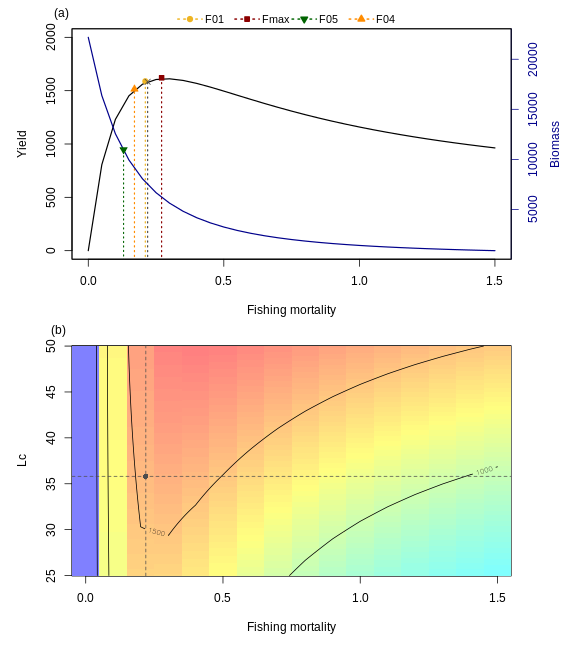Please note that the resolution of the $$L_c$$ and F changes is quite low and the range quite narrow due to the limitations in computation time of the vignette format. The results indicate that the fishing mortality of this example (F = 0.22) is smaller than the maximum fishing mortality ($$F_{max} =$$ 0.27), which confirms the indication of the exploitation rate (E = 0.44). The prediction plot shows that the yield could be increased when fishing mortality and mesh size is increased. The units are grams per recruit.

## Summary

For management purposes, fish stock assessments are mainly conducted for single species or stocks, which describe the management units of a population. There is much to be gained from multi-species and ecosystem models, but data requirements and complexity make them often unsuitable for deriving management advice. For data-poor fisheries, a traditional fish stock assessment solely based on length-frequency (LFQ) data of one year (as presented here) is particularly useful. LFQ data comes with many advantages over long time series of catch and effort or catch-at-age data [@Mildenberger2016]. In this exercise, the exploitation rate and results of the yield per recruit models indicate sustainable exploitation. The exploration of stock status and fisheries characteristics can of course be extended, but go beyond the scope of this tutorial, which is thought to help getting started with the length-based assessment of the TropFishR package. Further details about functions and their arguments can be found in the help files of the functions (help(...) or ?.., where the dots refer to any function of the package). Also the two publications by @Mildenberger2016 and by @Taylor2016 provide more details about the functionality and context of the package.